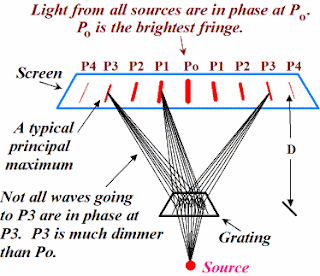## Pages

### 4.2 Diffraction grating

A diffraction grating consists of a large number of fine, equidistant, closely spaced parallel lines of equal width, ruled on polished metal.Sample Diffraction Grating
The diagram below is an experimental setup
Below is here's what happens in the diffraction grating,

Enlarged version, where only two slits are shown,

The diffraction grating formula for the principle maxima is as follows,
$$dsin(\theta_{n})=n\lambda$$
Where, $d$=distance between the slits
$\theta$= angle to the principle maxima
$n$= integer number to next bright fringe
$\lambda$= wavelength of the incident lightpiccourtesy:http://www.pstcc.edu/nbs/WebPhysics
Here's an easy to understand derivation for diffraction grating,

Examples: A monochromatic light of wavelength $5.0\times 10^{-7}m$ is incident normally on a plane diffraction grating and second-order bright lights emerge at angles of $30^{0}$ to the normal to the grating. Calculate the number of lines per millimeter of the grating. Ans: $500$